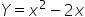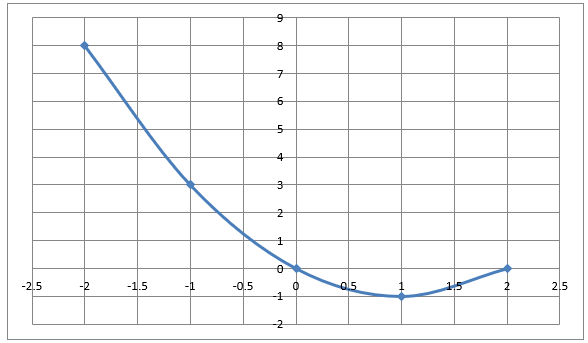Maths-
General
Easy

Question

# Determine whether the following functions are linear or non-linear and explain how you know.## The correct answer is: 8,3,0,-1,0

### Hint:In simpler terms, a linear function is one whose graph is a straight line when plotted on the xy plane, whereas a non- linear function can be any curve in the xy plane. So we will make a table of different values of x and corresponding values of y and plot the ordered pairs in the xy plane to get a graph.Step by step solution:The given equation isFirst we make a table of points satisfying the equation.Putting x = 0 in the above equation, we will get, y = 0Similarly, putting x = 1, in the above equation, we get y = -1Continuing this way, we haveFor x = -1, we get y = 3For x = 2, we get y = 0For x = -2, we get y = 8Making a table of all these points, we have X -2 -1 0 1 2 Y 8 3 0 -1 0 Now we plot these points on the graph.We join these points with a smooth curve to get the curveWe can clearly see that the graph is not a straight line.Hence, the given function is not linear.Note:There are other ways to determine if a given equation is linear or not. If an equation can be written in the form y = ax + b, where a and b are constants, then the function is linear. Another way to check if an equation is linear is by checking the highest degree of a term in the equation. If the highest degree of any term in the equation is 1, then the equation is linear, and if the degree of any term is greater than 1, then the equation is non-linear.#### With Turito Foundation.#### Get an Expert Advice From Turito.